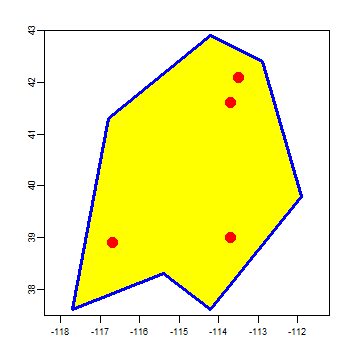# Vector data¶

## Introduction¶

The `terra` package defines a set of classes to represent spatial data. A class defines a particular data type. The `data.frame` is an example of a class. Any particular `data.frame` you create is an object (instantiation) of that class.

The main reason for defining classes is to create a standard representation of a particular data type to make it easier to write functions (known as “methods”) for them. See Hadley Wickham’s Advanced R or John Chambers’ Software for data analysis for a detailed discussion of the use of classes in R.

`terra` introduces a number of classes with names that start with `Spat`. For vector data, the relevant class is `SpatVector`. These classes represent geometries as well as attributes (variables) describing the geometries.

It is possible to create `SpatVector` objects from scratch with R code. This is very useful when creating small self contained example to illustrate something, for example to ask a question about how to do a particular operation without needing to give access to the real data you are using (which is always cumbersome). It is also frequently done when using coordinates that were obtained with a GPS. But in most other cases, you will read these from a file or database, see Chapter 5 for examples.

To get started, let’s make some SpatVector objects from scratch anyway, using the same data as were used in the previous chapter.

## Points¶

```longitude <- c(-116.7, -120.4, -116.7, -113.5, -115.5, -120.8, -119.5, -113.7, -113.7, -110.7)
latitude <- c(45.3, 42.6, 38.9, 42.1, 35.7, 38.9, 36.2, 39, 41.6, 36.9)
lonlat <- cbind(longitude, latitude)
```

Now create a `SpatVector` object. First load the `terra` package from the library. If this command fails with `Error in library(terra) : there is no package called ‘terra’`, then you need to install the package first, with `install.packages("terra")`

```library(terra)
pts <- vect(lonlat)
```

Let’s check what kind of object `pts` is.

```class (pts)
##  "SpatVector"
## attr(,"package")
##  "terra"
```

And what is inside of it

```pts
##  class       : SpatVector
##  geometry    : points
##  dimensions  : 10, 0  (geometries, attributes)
##  extent      : -120.8, -110.7, 35.7, 45.3  (xmin, xmax, ymin, ymax)
##  coord. ref. :
geom(pts)
##       geom part      x    y hole
##  [1,]    1    1 -116.7 45.3    0
##  [2,]    2    1 -120.4 42.6    0
##  [3,]    3    1 -116.7 38.9    0
##  [4,]    4    1 -113.5 42.1    0
##  [5,]    5    1 -115.5 35.7    0
##  [6,]    6    1 -120.8 38.9    0
##  [7,]    7    1 -119.5 36.2    0
##  [8,]    8    1 -113.7 39.0    0
##  [9,]    9    1 -113.7 41.6    0
## [10,]   10    1 -110.7 36.9    0
```

So we see that the object has the coordinates we supplied, but also an `extent`. This spatial extent was computed from the coordinates. There is also a coordinate reference system (“CRS”, discussed in more detail later). We did not provide the CRS when we created `pts`. That is not good, so let’s recreate the object, and now provide a CRS.

```crdref <- "+proj=longlat +datum=WGS84"
pts <- vect(lonlat, crs=crdref)
pts
##  class       : SpatVector
##  geometry    : points
##  dimensions  : 10, 0  (geometries, attributes)
##  extent      : -120.8, -110.7, 35.7, 45.3  (xmin, xmax, ymin, ymax)
##  coord. ref. : +proj=longlat +datum=WGS84 +no_defs
crs(pts)
##  "GEOGCRS[\"unknown\",\n    DATUM[\"World Geodetic System 1984\",\n        ELLIPSOID[\"WGS 84\",6378137,298.257223563,\n            LENGTHUNIT[\"metre\",1]],\n        ID[\"EPSG\",6326]],\n    PRIMEM[\"Greenwich\",0,\n        ANGLEUNIT[\"degree\",0.0174532925199433],\n        ID[\"EPSG\",8901]],\n    CS[ellipsoidal,2],\n        AXIS[\"longitude\",east,\n            ORDER,\n            ANGLEUNIT[\"degree\",0.0174532925199433,\n                ID[\"EPSG\",9122]]],\n        AXIS[\"latitude\",north,\n            ORDER,\n            ANGLEUNIT[\"degree\",0.0174532925199433,\n                ID[\"EPSG\",9122]]]]"
```

We can add attributes (variables) to the `SpatVector` object. First we need a `data.frame` with the same number of rows as there are geometries.

```# Generate random precipitation values, same quantity as points
precipvalue <- runif(nrow(lonlat), min=0, max=100)
df <- data.frame(ID=1:nrow(lonlat), precip=precipvalue)
```

Combine the `SpatVector` with the `data.frame`.

```ptv <- vect(lonlat, atts=df, crs=crdref)
```

To see what is inside:

```ptv
##  class       : SpatVector
##  geometry    : points
##  dimensions  : 10, 2  (geometries, attributes)
##  extent      : -120.8, -110.7, 35.7, 45.3  (xmin, xmax, ymin, ymax)
##  coord. ref. : +proj=longlat +datum=WGS84 +no_defs
##  names       :    ID precip
##  type        : <num>  <num>
##  values      :     1  6.179
##                    2   20.6
##                    3  17.66
```

## Lines and polygons¶

Making a `SpatVector` of points was easy. Making a `SpatVector` of lines or polygons is a bit more complex, but stil relatively straightforward.

```lon <- c(-116.8, -114.2, -112.9, -111.9, -114.2, -115.4, -117.7)
lat <- c(41.3, 42.9, 42.4, 39.8, 37.6, 38.3, 37.6)
lonlat <- cbind(id=1, part=1, lon, lat)
lonlat
##      id part    lon  lat
## [1,]  1    1 -116.8 41.3
## [2,]  1    1 -114.2 42.9
## [3,]  1    1 -112.9 42.4
## [4,]  1    1 -111.9 39.8
## [5,]  1    1 -114.2 37.6
## [6,]  1    1 -115.4 38.3
## [7,]  1    1 -117.7 37.6
lns <- vect(lonlat, type="lines", crs=crdref)
lns
##  class       : SpatVector
##  geometry    : lines
##  dimensions  : 1, 0  (geometries, attributes)
##  extent      : -117.7, -111.9, 37.6, 42.9  (xmin, xmax, ymin, ymax)
##  coord. ref. : +proj=longlat +datum=WGS84 +no_defs
```
```pols <- vect(lonlat, type="polygons", crs=crdref)
pols
##  class       : SpatVector
##  geometry    : polygons
##  dimensions  : 1, 0  (geometries, attributes)
##  extent      : -117.7, -111.9, 37.6, 42.9  (xmin, xmax, ymin, ymax)
##  coord. ref. : +proj=longlat +datum=WGS84 +no_defs
```

Behind the scenes the class deals with the complexity of accommodating for the possibility of multiple polygons, each consisting of multiple sub-polygons, some of which may be “holes”. You do not need to understand how these structures are organized. The main take home message is that a `SpatVector` stores geometries (coordinates), the name of the coordinate reference system, and attributes.

We can make use `plot` to make a map.

```plot(pols, las=1)